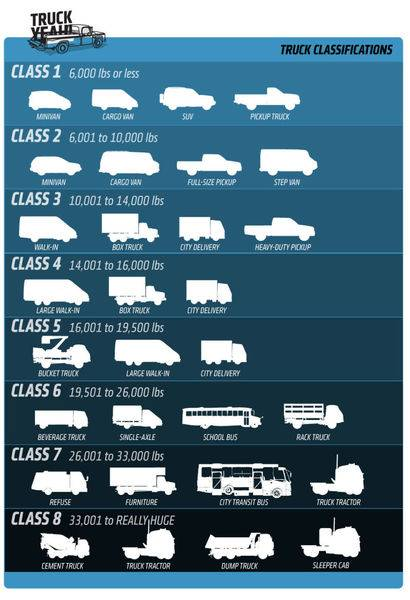What would the torque on a driveshaft be for a 8 ton vehicle in park on a 16% grade? Tire radius is 19". Axle ratio it 7.1.

berkeman
Mentor
What would the torque on a driveshaft be for a 8 ton vehicle in park on a 16% grade? Tire radius is 19". Axle ratio it 7.1.
Welcome to the PF.

Is this question for schoolwork? What is the context of the question?

Welcome to the PF.

Is this question for schoolwork? What is the context of the question?
Trying to determine the loads on an output shaft of a transmission when parked on a grade with different axle ratios. Not for school work. Just general knowledge.

•berkeman
jack action
Gold Member
If the rear wheels support the entire load caused by the slope, the force acting at the rear tires is ##F_w = W\sin\theta##, where ##W## is the weight of the vehicle and ##\theta## is the angle of the slope (reference). ##100\tan\theta = \%slope## to find the angle of the slope (reference).

The wheel torque produced is ##T_w = F_w r##, where ##r## is the tire radius.

The gear ratio reduces the torque seen by the driveshaft, so ##T_d = \frac{T_w}{GR}##, where ##GR## is the axle gear ratio.

##\theta = \arctan\frac{16}{100} = 9.1°##

##F_w = (16000\ lb)\sin9.1° = 2530\ lb##

##T_w = (2530.5\ lb) * (1.583\ ft) = 4006\ lb.ft##

##T_d = \frac{4006\ lb.ft}{7} = 572\ lb.ft##

If the rear wheels support the entire load caused by the slope, the force acting at the rear tires is ##F_w = W\sin\theta##, where ##W## is the weight of the vehicle and ##\theta## is the angle of the slope (reference). ##100\tan\theta = \%slope## to find the angle of the slope (reference).

The wheel torque produced is ##T_w = F_w r##, where ##r## is the tire radius.

The gear ratio reduces the torque seen by the driveshaft, so ##T_d = \frac{T_w}{GR}##, where ##GR## is the axle gear ratio.

##\theta = \arctan\frac{16}{100} = 9.1°##

##F_w = (16000\ lb)\sin9.1° = 2530\ lb##

##T_w = (2530.5\ lb) * (1.583\ ft) = 4006\ lb.ft##

##T_d = \frac{4006\ lb.ft}{7} = 572\ lb.ft##

Shouldn't this include the acceleration of gravity?
F_w = 16000 Lbs* 32.174 ft/s2

Shouldn't this include the acceleration of gravity?
F_w = 16000 Lbs* 32.174 ft/s2

pounds is a unit for weight not mass, already includes gravity.

•jack action
Baluncore
I used SI units.
Mass = 8000 kg.
Force due to gravity = 8000 * 9.8 = 78400 newton.
Wheel radius is 39” = conveniently 1 metre.
16% grade = 9.09 deg; Sin(9.09°) = 0.158
7:1 axle ratio.
Drive shaft torque = 1m * 78400N * 0.158 / 7 = 1769.5 Nm
1769.5 Nm = 1305.1 ft.lbs
This is quite different to jack action's 572. ft.lb

Last edited:
jack action
Gold Member
I used SI units.
Mass = 8000 kg.
Force due to gravity = 8000 * 9.8 = 78400 newton.
Wheel radius is 39” = conveniently 1 metre.
16% grade = 9.09 deg; Sin(9.09°) = 0.158
7:1 axle ratio.
Drive shaft torque = 1m * 78400N * 0.158 / 7 = 1769.5 Nm
1769.5 Nm = 1305.1 ft.lbs
This is quite different to jack action's 572. ft.lb
That is because we don't use the same numbers:
• I use 1 ton = 2000 lb and you use 1 tonne = 1000 kg;
• I use a wheel radius of 19" and you use a wheel radius of 39".
Other than that, everything is the same!•Baluncore
Baluncore
Fixing the radius to 19” and using a mass of 8 Short Tons.
Mass 8 short ton = 7257.5 kg.
Force due to gravity = 7257.5 * 9.8 = 71123.5 newton.
Wheel radius is 19” = inconveniently 0.4826 metre.
16% grade = 9.09 deg; Sin(9.09°) = 0.158
7:1 axle ratio.
Drive shaft torque = 0.4826 * 71123.5 * 0.158 / 7 = 774.75 Nm
774.75 Nm = 571.5 ft.lbs
We agree.

In Australia the standard Ton was a Long Ton = 1016.05 kg = 2240 lbs.
In 1966 that was replaced by the metric Tonne = 1000 kg = 2205 lbs.

Are vehicle weights in the USA always specified in Short Tons = 907.18 kg = 2000 lbs ?

jack action
Gold Member
Are vehicle weights in the USA always specified in Short Tons = 907.18 kg = 2000 lbs ?
https://en.wikipedia.org/wiki/Short_ton

I'm in Canada and a ton has always been 2000 lb around here. But we're with the SI system as well, so this is not a usual unit for us nowadays (unless we have to do business with our neighbor).

As for truck classification, it is done in pounds, so no problem there:•Baluncore